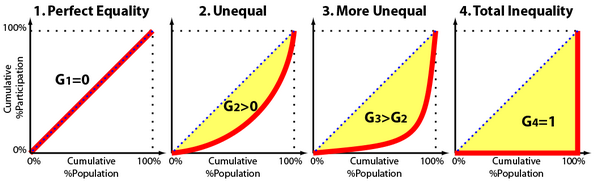# Series Exercises¶

To get accustomed to Series, let’s explore some data on the wealth of 10 randomly selected countries. Data below presents the GDP per capita for these countries in 2008.

## Exercise 1¶

Use the code below to get started:

import pandas as pd
gdppercap = pd.Series([34605, 34493, 12393, 44200, 10041,
58138, 4709, 49284, 10109, 42536],
index=['Bahrain', 'Belgium', 'Bulgaria',
'Ireland', 'Macedonia', 'Norway',
'Paraguay', 'Singapore',
'South Africa', 'Switzerland']
)


## Exercise 2¶

Find the mean, median, minimum and maximum values of GDP per capita in this data.

### Exercise 3¶

Programmatically, determine which country in our data has the highest income per capita, and which has the lowest income per capita.

(Obviously, this is easier to do by just looking at the data, but that’s only because this dataset is very small. With a real dataset, you would need to do it with code, so please write code to accomplish this task.)

Hint: Country names form the index for this Series, so to get country names you’ll need to access the index.

:

import pandas as pd
gdppercap = pd.Series([34605, 34493, 12393, 44200, 10041,
58138, 4709, 49284, 10109, 42536],
index=['Bahrain', 'Belgium', 'Bulgaria',
'Ireland', 'Macedonia', 'Norway',
'Paraguay', 'Singapore',
'South Africa', 'Switzerland']
)



### Exercise 4¶

Get Python to print out the names of all the countries that have GDP per capitas less than \$20,000.

### Exercise 5¶

Get Python to print out the GDP per capita of Switzerland.

### Exercise 6¶

One frequntly used measure of inequality is the Gini Coefficient. The Gini Coefficient takes on a value of 1 when the distribution of some variable is maximally unequal across a population, and a value of 0 when it is evenly distributed. We will calculate the Gini Coefficient for income inequality in our data.

To visualize the Gini Coefficient, we plot the cumulative share of the population (ordered from poorest to richest) on the x-axis, and cumulative share of income earned by that group on the y-axis. The Gini Coefficient is then defined as

$\frac{A}{A + B}$

, where the areas A and B are labeled below:If income is evenly distributed, then the poorest 20% of a population will also have 20% of the wealth; the poorest 40% will have 40% of the wealth, and so forth, resulting in a perfect 45 degree line. In this situation, there is no area between the 45% line and the actual income distribution, so $$A=0$$, and the Gini Coefficient is 0.

If, by contrast, the top 10% of people hold all the wealth in a country, then there will be no wealth for the poorest 90% of people, then wealth will jump up at the far right side of the graph. This will generate a very large gap between the 45% line and actual income for most of the graph, generating a large value for the area $$A$$, creating a very high Gini Coefficient.

To illustrate, here are a few different Gini plots. These come from someone studying inequality of participation, so to adapt this to our study of income, just imagine the y-axis plots share of income):For discrete data, the Gini Coefficient can be calculated with the following formula:

$\frac{2 \sum_{i=1}^n i y_i}{n \sum_{i=1}^n y_i} -\frac{n+1}{n}$

Where $$i$$ is each country’s rank ordering from poorest to richest, and $$y_i$$ is the income of country $$i$$.

Using this formula, calculate the Gini coefficient for our income data.

HINT: Be careful with 0-indexing! Python counts from 0, but mathematical formulas count from 1!

HINT 2: I’m gonna make you calculate Gini coefficients again later, so maybe you should write a function to do this and make life easier later?

### Exercise 7¶

The result we just generated offers a snap-shot of inequality for this subset of countries. But what are the dynamics of inequality for these countries?

There is an idea in economics called the “convergence hypothesis”, which argues that poorer countries are likely to grow faster, and as a result global inequality is likely to decline. Economists advocating for this hypothesis pointed out that while rich countries had to invent new technologies in order to grow, many poor countries simply had to take advantage of innovations already developed by rich countries.

To test this hypothesis, let’s do a small analysis of the dynamics of income inequality in our sample. Create the following Series in your Python session, which provides the average growth rate of GDP per capita for all the countries in our sample from 2000 to 2018.

avg_growth = pd.Series([-0.29768835, 0.980299584, 4.52991925,
3.686556736, 2.621416804, 0.775132075,
2.015489468, 3.345793635, 1.349993318,
0.982775018],
index=['Bahrain', 'Belgium', 'Bulgaria',
'Ireland', 'Macedonia', 'Norway',
'Paraguay', 'Singapore',
'South Africa', 'Switzerland']
)


Using this data on average growth rates in GDP per capita, and assuming growth rates from 2000 to 2018 continue into the future, estimate what our Gini Coefficient may look like in 2025 (remembering that income in our data is from 2008, so we’re extrapolating ahead 17 years)?

Hint: the formula for compound growth (i.e. value of something growing at a rate of x percent for $$t$$ periods) is:

$future\_value = current\_value * (1 + \frac{percentage\_growth\_rate}{100})^t$

### Exercise 8¶

Interpret your result – does it seem to imply that we are seeing covergence or not?

After you’re done, you can see a more systematic version of this analysis here!

### Absolutely positively need the solutions?¶

Don’t use this link until you’ve really, really spent time struggling with your code! Doing so only results in you cheating yourself.